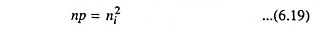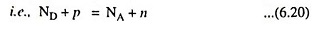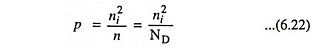## Charge Densities in Extrinsic Semiconductor:

Charge Densities in Extrinsic Semiconductor – Equation (6.19) provides relationship between the electron and hole concentrations n and p respectively.If ND is the concentration of donor atoms, there will be ND immobile positive charges per unit volume contributed by donor ions as the donor atoms are ionized. The positive charge density will be ND + p. Similarly as the acceptor atoms are ionized at room temperature there will he NA immobile negative charges per unit volume and total negative charge density will be NA + n, where NA is the concentration of acceptor atoms. Since the semiconductor is electrically neutral, the magnitude of positive charge-density will be equal to the magnitude of negative charge-density.In N-type semiconductors there is no acceptor doping i.e., NA = 0. Also the number of electrons is much greater than the number of holes i.e., n ≫ p.i.e., in N-type semiconductors, the free electron concentration is approximately equal to the density of donor atoms.

Now the concentration of holes in N-type semiconductors will be given by the equationSimilarly in case of P-type semiconductors

Scroll to Top